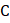# A solid sphere of mass, radiusand having moment of inertia about an axis passing through the centre of mass as I, is recast into a disc of thickness, whose moment of inertia about an axis passing through its edge and perpendicular to its plane remains I. Then, radius of the disc will be a)b)c)d)## Question ID - 100179 :- A solid sphere of mass, radiusand having moment of inertia about an axis passing through the centre of mass as I, is recast into a disc of thickness, whose moment of inertia about an axis passing through its edge and perpendicular to its plane remains I. Then, radius of the disc will be a)b)c)d)3537

 (a)An object initially at rest explodes into three fragmentsand. The momentum ofisand that ofiswhereis anumber.The momentum ofwill be a) (1+in a direction making anglewith that ofb) (1+in a direction making anglewith that ofc)in a direction making anglewith that ofd)in a direction making anglewith that of